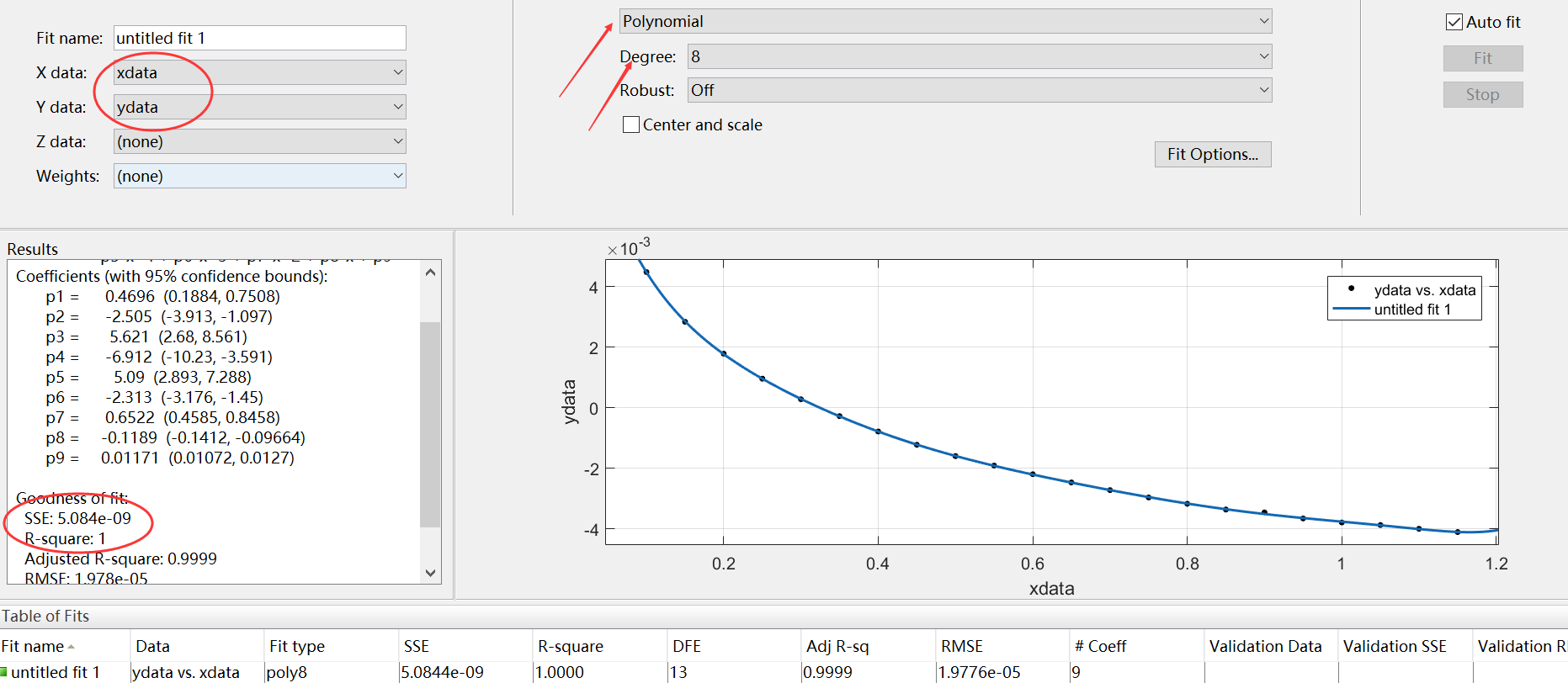• matlab函数曲线拟合代码Geomech IITR 用于标准井解释和地质力学建模的MATLAB工具 一般特征 简单的GUI平台进行测井分析 电缆日志数据的可视化：加载和绘制电缆日志以可视化日志数据 综合日志建模器：使用我们的方程...
• 通过构造一个新的、精确的和通用的保角映射,利用Muskhelishvili复势法研究了任意自然数次幂函数型曲线裂纹的平面弹性问题,给出了远处受单向拉伸载荷下裂纹尖端Ⅰ型和Ⅱ型应力强度因子的一般解.当幂次取不同的...
• plot (on log-log axes) the empirical distribution along with the fitted power-law distribution
• 函数：p = polyfit(x,y,n)其中：x是已知的离散数据点的横坐标，y是已知离散数据点的纵坐标，n为需要拟合的最高次幂，由我们给定，运用不同的多项式进行拟合，返回值p从左到右是高到低的多项式p(x)的系数，长度是...

polyfit：最小二乘多项式曲线拟合
已知离散点上的数据集，即已知在点集上的函数值，构造一个解析函数（其图形为一曲线）使在原离散点上尽可能接近给定的值。
函数：p = polyfit(x,y,n)
其中：x是已知的离散数据点的横坐标，y是已知离散数据点的纵坐标，
n为需要拟合的最高次幂，由我们给定，运用不同的多项式进行拟合，
返回值p从左到右是高次到低次的多项式p(x)的系数，长度是n+1
p(x)=p1xn+p2xn−1+...+pnx+pn+1
函数：y=polyval(p,x);      %根据拟合的函数得出x对应的因变量y的值
多项式n的阶数的确定：
可以用MATLAB的拟合工具箱，cftool进行选择
在MATLAB主窗口中输入 cftool 回车 ，会弹出拟合工具箱界面选择拟合的参数，在右上角选择拟合方式为“Polynomial”，然后通过选择不同的degree，看右下角看离散点是否落在拟合曲线是以及Results里的SSE(方差)和R-square（相关系数），上图中的拟合，可以看到离散点都落在了拟合线上，并且相关系数为1，方差在-9的数量级上，拟合良好。


展开全文• matlab函数曲线拟合代码回声密度测量 这些脚本和数据说明了针对脉冲响应信号中的时间回波密度的“排序中心时间”度量，该度量在以下论文中提出： Helena Peic Tukuljac，Ville Pulkki，Hannes Gamper，Keith Godin...
• 说明：大于一的级数与陡峭程度正相关，小于一的级数相当于曲线顺时针旋转90°，底数增减的量决定在横轴的平移。 <!DOCTYPE html> <html lang="utf-8"> <meta ...

说明：大于一的幂级数与陡峭程度正相关，小于一的幂级数相当于曲线顺时针旋转90°，底数增减的量决定在横轴的平移。<!DOCTYPE html>
<html lang="utf-8">
<meta http-equiv="Content-Type" content="text/html; charset=utf-8"/>
<title>绘制二次三次曲线多次曲线</title>

<canvas id="myCanvus" width="1300px" height="640px" style="border:1px dashed black;">
出现文字表示你的浏览器不支持HTML5
</canvas>
</body>
</html>
<script type="text/javascript">
<!--
function draw(){
var canvas=document.getElementById("myCanvus");
var canvasWidth=1300;
var canvasHeight=640;

var context=canvas.getContext("2d");

context.fillStyle = "white";
context.fillRect(0, 0, canvasWidth, canvasHeight);

context.strokeStyle = "black";
context.fillStyle = "black";

// 进行坐标变换：把原点放在左下角，东方为X轴正向，北方为Y轴正向
var offsetY=320;// Y向偏移值，正值向上偏，用来画坐标轴
var offsetX=650;// X向偏移值，正值向右偏，用来画坐标轴

context.save();
context.translate(0+offsetX,canvasHeight-offsetY);

drawAxisXText(context);// 文字和线分开画比较好处理
drawAxisYText(context);
drawTitleText(context);

context.scale(-1,1);

drawAxisX(context);
drawAxisY(context);
drawCurve(context);

context.restore();
}

function drawTitleText(ctx){
ctx.lineWidth=0.5;
ctx.strokeStyle='navy';
ctx.fillStyle='navy';

var x=350;
var y=-250;

// 写文字
ctx.fillText("y=x^2 红色",x,y);
ctx.fillText("y=(x-3)^3 绿色",x,y+20);
ctx.fillText("y=(x-5)^4 黄色",x,y+40);
ctx.fillText("y=(x-7)^5 青柠色",x,y+60);
ctx.fillText("y=(x+3)^0.5 紫色",x,y+80);
ctx.fillText("y=(x+5)^0.33 栗色",x,y+100);

ctx.fillText("  绘制:逆火狂飙",x+170,y+30);
}

function drawCurve(ctx){
var cds=[{}];
var cds1=[{}];
var cds2=[{}];
var cds3=[{}];
var cds4=[{}];
var cds5=[{}];
var cds6=[{}];

var x,y,arr;
for(x=-13;x<=13;x+=0.01){
y=Math.pow(x,2);//
arr={"x":x,"y":y};
cds.push(arr);

y=Math.pow(x-3,3);//
arr={"x":x,"y":y};
cds1.push(arr);

y=Math.pow(x-5,4);//
arr={"x":x,"y":y};
cds2.push(arr);

y=Math.pow(x-7,5);//
arr={"x":x,"y":y};
cds3.push(arr);

y=Math.pow(x+3,1/2);//
arr={"x":x,"y":y};
cds4.push(arr);

y=Math.pow(x+5,1/3);//
arr={"x":x,"y":y};
cds5.push(arr);
}

paintCurve(ctx,"red",cds);
paintCurve(ctx,"green",cds1);
paintCurve(ctx,"yellow",cds2);
paintCurve(ctx,"lime",cds3);
paintCurve(ctx,"purple",cds4);
paintCurve(ctx,"maroon",cds5);
//paintCurve(ctx,"maroon",cds6);*/

/*var ymax=-1000,ymin=1000,xmax,xmin;
for(var i=0; i<cds.length; i++){
// 求y最大值
if(cds[i].x<0 && cds[i].y>ymax){
ymax=cds[i].y;
xmax=cds[i].x;
}

// 求y最小值
if(cds[i].x>=0 && cds[i].y<ymin){
ymin=cds[i].y;
xmin=cds[i].x;
}
}

console.log("ymin="+ymin+" xmin="+xmin+" ymax="+ymax+" ymin="+ymin+" xmax="+xmax);
var SU=50;// Scale Unit
// 极大值
ctx.beginPath();
ctx.moveTo(xmax*SU,ymax*5-5);
ctx.lineTo(xmax*SU,ymax*5+5);

ctx.save();
ctx.scale(1,-1);
ctx.fillText("ymax="+cutShort(ymax.toString(),8),xmax*SU,-ymax*5);
ctx.restore();

ctx.stroke();
ctx.closePath();

// 极小值
ctx.beginPath();
ctx.moveTo(xmin*SU,ymin*5-5);
ctx.lineTo(xmin*SU,ymin*5+5);

ctx.save();
ctx.scale(1,-1);
ctx.fillText("ymin="+ymin,xmin*SU,-ymin*5);
ctx.restore();

ctx.stroke();
ctx.closePath();*/

}

function paintCurve(ctx,color,cds){
var SU=50;// Scale Unit

ctx.strokeStyle = color;
ctx.beginPath();
for(var i=0; i<cds.length; i++){
ctx.lineTo(cds[i].x*SU,cds[i].y*SU);// 注意y轴比例
}
ctx.stroke();
ctx.closePath();
}

function drawAxisX(ctx){
ctx.save();

ctx.lineWidth=0.5;
ctx.strokeStyle='navy';
ctx.fillStyle='navy';

var start=-650;
var end=650;

// 画轴
ctx.beginPath();
ctx.moveTo(start, 0);
ctx.lineTo(end, 0);
ctx.stroke();
ctx.closePath();

// 画箭头
ctx.beginPath();
ctx.lineTo(end, 0);
ctx.stroke();
ctx.closePath();

// 画刻度
var x,y;
y=5;
for(x=start;x<end;x+=50){
ctx.beginPath();
ctx.moveTo(x, 0);
ctx.lineTo(x, y);

ctx.stroke();
ctx.closePath();
}

ctx.restore();
}

function drawAxisXText(ctx){
ctx.lineWidth=0.5;
ctx.strokeStyle='navy';
ctx.fillStyle='navy';

var start=-650;
var end=650;

// 写文字
var x,y=5;
for(x=start;x<end;x+=50){
ctx.fillText(x/50,x,y+10);
}
}

function drawAxisY(ctx){
ctx.save();

ctx.lineWidth=0.5;
ctx.strokeStyle='navy';
ctx.fillStyle='navy';

var start=-300;
var end=300;

// 画轴
ctx.beginPath();
ctx.moveTo(0, start);
ctx.lineTo(0, end);
ctx.stroke();
ctx.closePath();

// 画箭头
ctx.beginPath();
ctx.lineTo(0, end);
ctx.stroke();
ctx.closePath();

// 画刻度
var x,y;
x=5;
for(y=start;y<end;y+=50){// 注意y轴比例
ctx.beginPath();
ctx.moveTo(x, y);
ctx.lineTo(0, y);

ctx.stroke();
ctx.closePath();
}
}

function drawAxisYText(ctx){
ctx.lineWidth=0.5;
ctx.strokeStyle='navy';
ctx.fillStyle='navy';

var start=-250;
var end=350;

// 写文字
var x=-19,y=5;
for(y=start;y<end;y+=50){

if(y!=0){
ctx.fillText(-y/50,x,y);// 注意y轴比例
}
}
}

return degree/180*Math.PI;
}

function cutShort(str,length){
if(str.length>length){
str=str.substr(0,length)+"...";
}

return str;
}
//-->
</script>本文转自张昺华-sky博客园博客，原文链接：http://www.cnblogs.com/xiandedanteng/p/8157169.html，如需转载请自行联系原作者


展开全文• 基于幂曲线映射的测量矩阵优化算法，王若乾，赵瑞珍，测量矩阵是压缩感知的基础，贯穿着整个采样、重构过程，其性能的优劣直接影响着采样数据的完整性和重构信号的精确性，起着关键性压缩感知
• 收集的问题： 如何用matlab来拟合律分布，怎样将拟合值和实际值...在MATLAB里，对数据进行拟合，在双对数坐标下，看数据是否符合律分布，求出指数，并绘出图形。-In MATLAB, the pairs of data fitting, in

收集的问题：
如何用matlab来拟合幂律分布，怎样将拟合值和实际值进行对比，放在一个图中，又如何检验实际数据是否符合拟合函数。如果不符合，如何来直接判断实际数据服从什么样的函数分布呢

在MATLAB里，对数据进行拟合，在双对数坐标下，看数据是否符合幂律分布，求出幂指数，并绘出图形。-In MATLAB, the pairs of data fitting, in double logarithmic coordinates to see whether the data meet the power-law distribution, find the power index, and draw the graph.以傅雷书信为例：（平移237个单位时间）
“>>”后是输入内容，行开头不含“>>”的是MATLAB运行的结果，“/”后是注释部分

>> x=[ ];  / 输入修正的书信间隔时间
>> y=[ ];  / 输入累积概率
>> loglog(x,y,'ko')  / 画出双对数下坐标图（k表示黑色，o表示圆圈）
>> hold on  / 保留刚才所画图表，以便继续在此图画出拟合直线
>>  a=polyfit(log(x),log(y),1)  / 求拟合直线的参数（一次项和常数项）

a =

-1.0700    5.9525

>> b=2.71828^5.9525  /  常数项转化

b =

384.7124

>> x=100:100000;  /  根据上面所作的图指定x的取值范围
>> y=b*x.^ -1.0700 ;  / 根据刚才的计算输入x和y的关系式
>> plot(x,y,'k')  / 在双对数坐标下画出拟合直线

上面程序即可得到我们需要的图形，图形的再编辑可以在Figure窗口下的Edit-Figure Properties里修改（颜色、线条粗细、坐标轴命名等）。
但是得注意的是，用这个plotfit函数不太能够用来拟合很复杂的函数，而只是用来拟合线性的、二维之类的，而用它来拟合幂律分布的曲线时，只能考虑先截取一部分的点，然后用这个函数去拟合。

MATLAB软件提供了基本的曲线拟合函数的命令．   多项式函数拟合：P=polyfit(x,y,n)   其中n表示多项式的最高阶数，x，y为将要拟合的数据，它是用数组的方式输入．输出参数P为拟合多项式 P(1)*X^N + P(2)*X^(N-1) +...+ P(N)*X + P(N+1).的系数  多项式在x处的值y可用下面程序计算．  y=polyval(P,x,m)   线性：m=1, 二次：m=2, …   polyfit的输出是一个多项式系数的行向量。为了计算在xi数据点的多项式值，调用MATLAB的函数polyval。   例：   x=0:0.1:1;   y=[-0.447 1.978 3.28 6.16 7.08 7.34 7.66 9.56 9.48 9.30 11.2];   A=polyfit(x,y,2)   Z=polyval(A,x);   Plot(x,y,’r*’,x,z,’b’)

polyfit不能保证你每次都能得到最优解，math的答案是使用数值计算。  个人认为，对于这种非线性的曲线，尽量不要使用ployfit, ployfit多项式抑合适合线性方程！！

用polyfit（）函数去拟合这么复杂的曲线不太合适，polyfit（）函数对于数据遵循多项式分布是比较好的，一般来说，利用polyfit（）函数拟合的阶数不要超过5阶。  如果是不需要得到拟合曲线的函数，只是把这些点利用一些光滑曲线连接，建议使用三次样条函数spline（）进行插值即可。


展开全文matlab 线性拟合
• 利用复变函数法，通过引入适当的保角变换，研究了裂纹面受剪切作用下无限大点群6一维六方准晶中函数型曲线裂纹的断裂行为，给出了曲线型裂纹在裂纹尖端处应力强度因子公式，得到了裂纹尖端处应力强度因子的解析解...
• 研究含函数类对称曲线裂纹平面弹性问题，与解决孔口问题类似，采用传统的复变函数保角映射法，给出适当的保角变换公式，将裂纹外的区域映射到一个复平面的单位圆内，得到了含函数类对称曲线裂纹尖端Ⅰ-Ⅱ型应力...python
• matlab的双曲线代码双曲变换 这是本文的补充材料： Arthur C. Tsai *，Michelle Liou *，Maria Simak和Philip E. Cheng。 关于双曲正态变换。 计算统计与数据分析，115：250–266，2017年。 Matlab脚本演示了双曲...
• 讨论了GM(1，1，tα)幂次时间项模型的曲线形状、发展系数以及指数间的关系，研究了非等间隔GM(1，1，tα)幂次时间项模型的参数空间．将平均相对洪差看成指数的函数，根据序列形状判断指数的范围，并利用粒子群...
• 幂次变换的基本表达式为：y=cxr+b  其中c、r均为正数。与对数变换相同，幂次变换将部分灰度区域映射到更宽的区域中。当r=1时，幂次变换转变为线性变换。  (1) 当r  (2) 当r>0时，变换函数曲线在正比函数下方...
幂次变换的基本表达式为：y=cx
r+b
其中c、r均为正数。与对数变换相同，幂次变换将部分灰度区域映射到更宽的区域中。当r=1时，幂次变换转变为线性变换。
(1)  当r<0时，变换函数曲线在正比函数上方。此时扩展低灰度级，压缩高灰度级，使图像变亮。这一点与对数变换十分相似。
(2)  当r>0时，变换函数曲线在正比函数下方。此时扩展高灰度级，压缩低灰度级，使图像变暗。
代码如下：

[cpp]
view plain
copy
print
?

/******************************************************************************      *   作用:     幂次变换函数 *   参数: pDst     输出图像的像素数组 *   参数: pSrc     原始图像的像素数组 *   参数: nWidth   原始图像宽度 *   参数: nHeight  原始图像高度 *   参数: b          控制参数,表示曲线的上下偏移量 *   参数: c          控制参数,表示曲线的弯曲程度 *   参数: r          控制参数,表示函数的幂次 *   备注: 此函数对于彩色图同样适用 ******************************************************************************/  int PowerTrans(BYTE* pDst, BYTE* pSrc, int nWidth, int nHeight, double b, double c, double r)  {      if (!pSrc || !pDst)      {          return EXIT_FAILURE;      }        // 映射表,用于256种灰度变换后的值      BYTE map;        // 保存运算后的临时值      double dTemp;      int i, j;      for (i = 0; i < 256; i++)      {          // 计算当前像素变换后的值          dTemp = c * pow(i / 255.0, r) * 255 + b;            // 如果超界则修改其值          if (dTemp < 0)              dTemp = 0.0;          else if (dTemp > 255)              dTemp = 255;            // 四舍五入          map[i] = int(dTemp + 0.5);      }        for (i = 0; i < nWidth * nHeight; i++)      {             for (j = 0; j < 4; j++)              pDst[i*4+j] = map[ pSrc[i*4+j] ];      }        return EXIT_SUCCESS;  }


展开全文图像处理 算法 byte 扩展 c
• 本文详细介绍了样条曲线的相关内容，以常用的三样条曲线为例，进行详细的推导，并提供了基于Opencv的代码和测试程序。opencv Spline
• l-曲线矩阵代码 标题 作者 日期 输出 降价促销 纳米级的功率定律等。 2015年8月23日，星期日 ...数据集和内容为： 纳米力在环境条件下的功率定律 ...Olukan），塞尔吉奥·桑托斯（Sergio ...律（原始和半处理的数
• 输入数据为：日期、渠道、第几日留存(x_value)、第几日留存率(y_value)【若拟合app整体留存曲线，则去掉渠道字段】 输出数据为：日期、渠道、a、b、lt【同上】 #!/usr/bin/python # -*- coding: utf-8 -*- ...python
• 这是你第一使用 Markdown编辑器 所展示的欢迎页。如果你想学习如何使用Markdown编辑器, 可以仔细阅读这篇文章，了解一下Markdown的基本语法知识。 新的改变 我们对Markdown编辑器进行了一些功能拓展与语法支持...
• 文章目录幂次变换核磁共振图像代码实现遇到问题附代码： 幂次变换 幂次变换，点运算的一种，运算公式为s=crγ，其中，c和γ是正常数。 当γ<1，此时扩展低灰度级，压缩高灰度级，在正比函数上方，使图像变亮； 当...
• 这个程序将能对女性的性感度作出图形描绘，并将其的重要参数转化为数字，如xlen/ylen即是程序提供给我们的衡量量。 从图中你可以看出，当这个比值处于0.45-0.55为是性感诱人。 采用VC6.0编写 内含源代码。
• excel 方程拟合 包含线性，指、函数，三阶贝塞尔绘图拟合曲线，贝塞尔（Bezier）求控制点
• 在接下来的部分中，通过最小二乘法估计回归曲线的参数w，使得回归曲线到样本点垂直距离（残差或误差）的平方和最小，即代价函数J(w)最小。 最小二乘法：主要思想就是选择未知参数，使得真实值和预测值之差的平方和...
• 一个采用Delphi编制的任意多项式曲线拟合类及其演示算例。有四种多项式可供选择：指数多项式、勒让德多项式、车比雪夫多项式、埃米特多项式，并实现了拟合后曲线的求导运算。可用于实验数据分析方面的编程。
• 我先通过Math.log(y,x)求出所有点的，然后再求这些的平均数，但是这得到的值有偏差，请各位大神给给建议，十万火急呀
• 空间域图像变换：图像反转，对数变换，幂次变换、分段线性变换 (s:现点值，r: 原点值) （一）图像反转： 这个无需多说，就是把黑变白，白变黑，拿八位灰度图像来说 表达式：s=255-r 作用：看清暗色图像中白色和...对数变换 图像翻转...